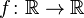# RANGE

In mathematics, the range of a function refers to either the codomain or the image of the function, depending upon usage. This ambiguity is illustrated by the function f that maps real numbers to real numbers with f(x) = x2. Some books say that range of this function is its codomain, the set of all real numbers, reflecting that the function is real-valued. These books call the actual output of the function the image. This is the current usage for range in computer science. Other books say that the range is the function’s image, the set of non-negative real numbers, reflecting that a number can be the output of this function if and only if it is a non-negative real number. In this case, the larger set containing the range is called the codomain. This usage is more common in modern mathematics.

Because of this ambiguity, it is a good idea to specify whether it is the image or the codomain being discussed.

## Examples

Let f be a function on the real numbersdefined by f(x) = 2x. This function takes as input any real number and outputs a real number two times the input. In this case, the codomain and the image are the same (i.e., the function is a surjection), so the range is unambiguous; it is the set of all real numbers.

In contrast, consider the functiondefined by f(x) = sin(x). If the word “range” is used in the first sense given above, we would say the range of f is the codomain, all real numbers; but since the output of the sine function is always between -1 and 1, “range” in the second sense would say the range is the image, the closed interval from -1 to 1.

## Formal definition

Standard mathematical notation allows a formal definition of range.

In the first sense, the range of a function must be specified; it is often assumed to be the set of all real numbers, and {y | there exists an x in the domain of f such that y = f(x)} is called the image of f.

In the second sense, the range of a function f is {y | there exists an x in the domain of f such that y = f(x)}. In this case, the codomain of f must be specified, but is often assumed to be the set of all real numbers.

In both cases, image f ⊆ range f ⊆ codomain f, with at least one of the containments being equality.